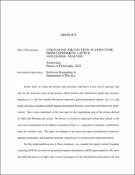## UNRAVELING THE NUCLEON 3D STRUCTURE FROM EXPERIMENT, LATTICE, AND GLOBAL ANALYSISIn this work, we study the nucleon spin structures and derive a new twist-3 partonic sum rule for the transverse spin of the proton, which involves the well-known quark spin structure function $g_T(x)$, the less-studied but known transverse gluon polarization density $\Delta G_T(x)$, and quark and gluon canonical orbital angular momentum densities associated with transverse polarization. This is the counterpart of the sum rule for the longitudinal spin of the proton derived by Jaffe and Manohar previously. We discuss in detail an important technicality related to the transverse polarization in the infinite momentum frame, i.e., separation of intrinsic contributions from the extrinsic ones. We apply our finding to the transverse-space distributions of partons, angular momentum, and magnetic moment, respectively, in a transversely polarized proton. For the experimental access to these structures, we consider the deeply virtual Compton scattering (DVCS) in twist-two generalized parton distribution (GPD) approximation. We show that different choices of light-cone vectors and gauges for the final photon polarization will lead to different higher-order kinematical corrections. The choice of light-cone vectors affects kinematic corrections at the twist-three level, accounting for the differences between the cross-section formulas in the literature. On the other hand, kinematical corrections from higher-twist GPDs eliminate the light-cone dependence at twist three. Those light-cone dependencies are studied systematically at JLab 12 GeV and future EIC kinematics. They serve as the intrinsic systematic uncertainties in extracting the Compton form factors. More importantly, they are also necessary for understanding cross-section measurements with higher-twist precision and to reconstruct higher-order Compton form factors. As DVCS is mostly sensitive to the quark structure, for the gluon, we also consider the near threshold photoproduction of heavy vector quarkonium off the proton. We show that the production amplitude can be factorized in terms of gluonic GPDs and the quarkonium distribution amplitude, similar to the high-energy limit. At the threshold, the threshold kinematics has a large skewness parameter $\xi$, leading to the dominance of the spin-2 contribution over higher-spin twist-2 operators. Thus, threshold production data are useful to extract the gluonic gravitational form factors, allowing studying the gluonic contributions to the quantum anomalous energy, mass radius, spin and mechanical pressure in the proton. We use the recent GlueX data on the $J/\psi$ photoproduction to illustrate the potential physics impact from the high-precision data from future JLab 12 GeV and EIC physics program. To put various constraints together and obtain the GPDs, we present the first global analysis of GPDs combing lattice quantum chromodynamics (QCD) calculations and experiment measurements including global parton distribution functions (PDFs), form factors (FFs) and DVCS measurements. Together with the constraints at zero skewness, we fit GPDs to global DVCS measurements from both the recent JLab and the earlier Hadron-Electron Ring Accelerator (HERA) experiments with two active quark flavors and leading order QCD evolution. With certain choices of empirical constraints, both sea and valence quark distributions are extracted with the combined inputs, and we present the quark distributions in the proton correspondingly. We also discuss how to extend the framework to accommodate more off-forward constraints beyond the small $\xi$ expansion, especially the lattice calculated GPDs.